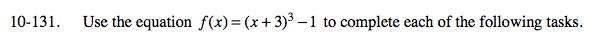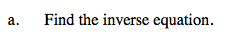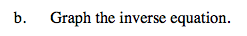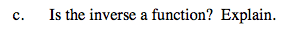### Home > A2C > Chapter Ch10 > Lesson 10.2.5 > Problem10-131

10-131.y = (x + 3)3 − 1 Switch x and y.
x = (y + 3)3 − 1
(x + 1) = (y + 3)3

$\sqrt{\textit{x}+1}=\textit{y}+3$

$\sqrt{\textit{x}+1}-3=\textit{y}$Reverse the x- and y-coordinates.Is there only one output (y) for each input (x)?